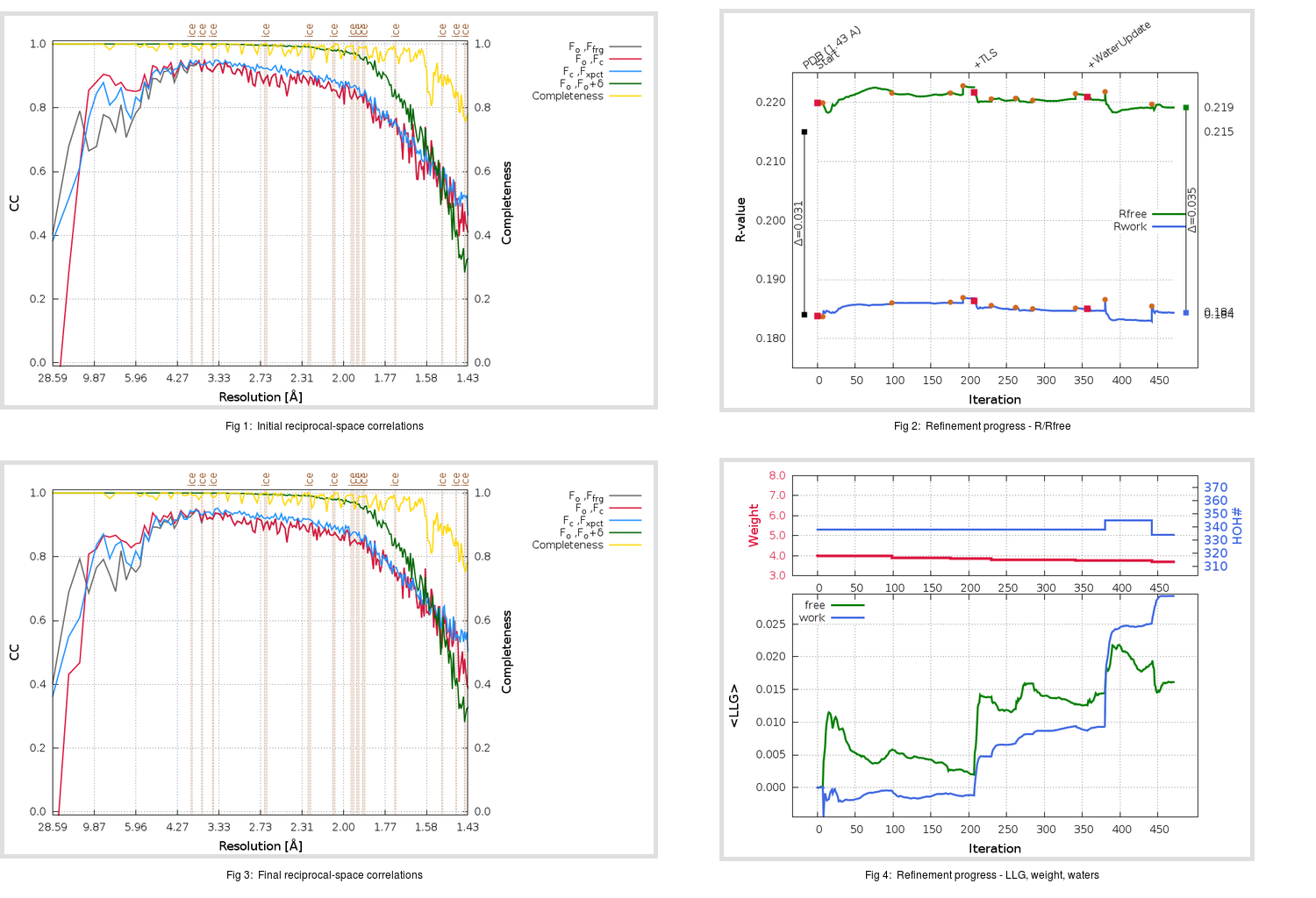Content:

```    Diffraction limits & principal axes of ellipsoid fitted to diffraction cut-off surface:
1.650         0.9997   0.0000  -0.0246       0.995 a* - 0.098 c*
1.551         0.0000   1.0000   0.0000       b*
1.374         0.0246   0.0000   0.9997       0.064 a* + 0.998 c*
```

## Deposited

` `
 Date deposited Date data collection Resolution R, Rfree 20200315 20200305 1.43 0.1820 0.2150

Molprobity (CCP4 7.0 version) summary:

```Ramachandran outliers =   0.00 %
favored =  97.68 %
Rotamer outliers      =   0.76 %
C-beta deviations     =     0
Clashscore            =   4.86
RMS(bonds)            =   0.0131
RMS(angles)           =   1.83
MolProbity score      =   1.32
Resolution            =   1.43
R-work                =   0.1820
R-free                =   0.2150
```

```Number of waters      =   338

<B> (all atoms) =   21.07 ( sd =    8.91 ) for       2735 non-hydrogen atoms
<B>   (protein) =   19.26 ( sd =    7.16 ) for       2370 non-hydrogen atoms
<B>     (water) =   33.22 ( sd =    9.94 ) for        338 non-hydrogen atoms
<B>    (others) =   26.16 ( sd =    9.84 ) for         27 non-hydrogen atoms

B min/max       (all non-hydrogen atoms) =    9.89 /   64.79
B min/max   (protein non-hydrogen atoms) =    9.89 /   58.85
B min/max     (water non-hydrogen atoms) =   11.26 /   64.79
B min/max     (other non-hydrogen atoms) =   21.60 /   34.00
```

## BUSTER (re-)refinement

` `

Molprobity (CCP4 7.0 version) summary:

```Ramachandran outliers =   0.00 %
favored =  98.68 %
Rotamer outliers      =   0.38 %
C-beta deviations     =     0
Clashscore            =   2.75
RMS(bonds)            =   0.0114
RMS(angles)           =   1.60
MolProbity score      =   1.06
Resolution            =   1.43
R-work                =   0.1845
R-free                =   0.2192
```

```Number of waters      =   334

<B> (all atoms) =   22.37 ( sd =    9.01 ) for       2731 non-hydrogen atoms
<B>   (protein) =   20.39 ( sd =    6.59 ) for       2370 non-hydrogen atoms
<B>     (water) =   35.80 ( sd =   11.47 ) for        334 non-hydrogen atoms
<B>    (others) =   28.40 ( sd =   10.35 ) for         27 non-hydrogen atoms

B min/max       (all non-hydrogen atoms) =    9.83 /   93.54
B min/max   (protein non-hydrogen atoms) =    9.83 /   58.19
B min/max     (water non-hydrogen atoms) =   13.04 /   93.54
B min/max     (other non-hydrogen atoms) =   24.29 /   36.20
```

Refinement progression:Results:

` `
 File Remark 5RF9_aB_refine.01_03_refine.pdb.gz exact refinement commands are in header 5RF9_aB_refine.01_03_refine.mtz.gz including original deposited data and several re-refinement map coefficients 5RF9_aB_refine.01_03_BUSTER_model.cif.gz including any non-standard compound restraints 5RF9_aB_refine.01_03_BUSTER_refln.cif.gz